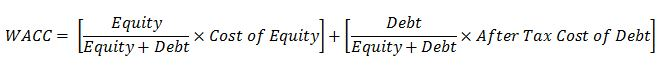## Key Financial Ratios Calculation ExampleAfter learning about key financial ratio from the previous article (Key Financial Ratios To Evaluate Companies
), this article will show an example based on the financial statements of CG Oil Company in 2014.

Figure 1 and Figure 2 are a company balance sheet and profit and loss account, respectively.Figure 1 – CG Oil Company Balance Sheet Continue reading

## Key Financial Ratios To Evaluate CompaniesIn order to evaluate a company’s financial health, there are some important ratios which will help investors get a clearer picture of companies.

Current Ratio – It is the current assets divided by the current liabilities in a company. This measures the ability of a company to pay current its liabilities over the next 12 months. Quick ratio should be at least more than 1. If its value is less than 1, it means that a company has insufficient cash to meet short term debts.

Current Ratio = Current Assets ÷ Current Liabilities Continue reading

## Weighted Average Cost Of Capital (WACC)Weighted Average Cost Of Capital (WACC) is a calculation of company cost of capital which is based on a weighted average between debt and equity. Its mathematical expression is listed below;WACC represents the minimum return on investment that can be a base line for a company to make a decision for new projects. Generally, companies will accept only projects that have returns more than WACC.

This example will use the previous information from Cost of Debt and Cost of Equity article to demonstrate WACC calculation.Continue reading

## Cost of EquityThe Cost of Equity is an investment return which a company offers its shareholders in compensation for their income stream and taking risk of investment with a company.

Cost of Equity which is based on the constant dividend growth model is mathematically expressed here.

## Cost of Equity = [Dn ÷ P] + G

Where;

Dn = dividend per share to be paid next year

P = average stock price in the year the latest dividend paid

G = expected growth of dividend on next year

A next year dividend is mathematically expressed like this.

## Dn = Dc × (1 + G)

Where;

Dc = dividend per share for present year

Therefore,